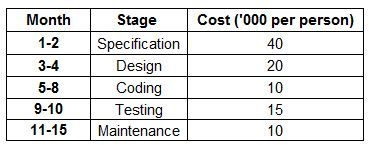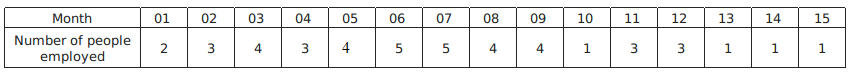### CAT 1996 Question Paper Question 59

Instructions

Answer the questions based on the following information. Mulayam Software Co., before selling a package to its clients, follows the given schedule.The number of people employed in each month is:Question 59

# Under the new technique, which stage of software development is most expensive for Mulayam Software Co.?

Solution

Total incurred cost for testing = $$(4+1)\times 15 = 75$$
Incurred cost for specification = $$(2+3) \times 40 = 200$$
Incurred cost for coding = $$(4+5+5) \times 10 = 140$$
Incurred cost for design = $$(3+4+5) \times 20 = 240$$

Hence design is most expensive for the company.

• All Quant CAT Formulas and shortcuts PDF
• 30+ CAT previous papers with solutions PDF